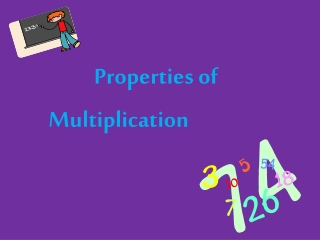DownloadDownload PresentationProperties of

# Properties of

Download Presentation## Properties of

- - - - - - - - - - - - - - - - - - - - - - - - - - - E N D - - - - - - - - - - - - - - - - - - - - - - - - - - -
##### Presentation Transcript

1. Properties of Multiplication

2. What are Properties? • Just like addition and subtraction, the operation of multiplication has different properties, or rules. • We have learned about some of these properties already! • Let’s review! 

3. Zero Property • When you multiply any number by zero, the product is always… ZERO!!!!! 0 x 6 =0 0 x 8 =0 0 x 4 =0 0 x 12 =0 Zeroes clear it out!

4. Identity Property • When you multiply a number by 1, the product will always be that number. • The number keeps its own identity—it doesn’t change! 1 x 5 = 5 1 x 9 = 9 1 x 2 = 2 1 x 7 = 7 Multiply by 1—it’s always the same!

5. Commutative Property • The Commutative Property of Multiplication is similar to the Commutative Property of Addition. • Inaddition, if you change the order of the addends the sum remains the same. • In multiplication, if you change the order of the factors the product remains the same. 9 x 7= 63 7 x 9 = 63 6 x 8 = 48 8 x 6 = 48 5 x 2 = 10 2 x 5 = 10

6. Associative Property • The associative property is also known as the grouping property. • It is similar to the associative property of addition. • You can change the grouping of the factors and the product will be the same! Let’s see what this looks like!

7. Let’s multiply 3 factors! There are different ways that you can find the product of 3 x 4 x 2 You can multiply 3 x 4 first. 3 x 4 = 12 Then multiply that product by 2. 12 x 2 = 24 You can group the factors differently and get the same product! 4 x 2 = 8 You can multiply 4 x 2 first. 8 x 3 = 24 Then multiply that product by 3.

8. Let’s try more! 5 x 2 x 2 = 4 x 5 x 1 = 2 x 6 x 2 = 3 x 3 x 4 =

9. Properties of Multiplication Zero Identity Commutative Associative Example Example Example Example Your Task: Complete the Tree Map with one example that illustrates each property of multiplication.